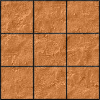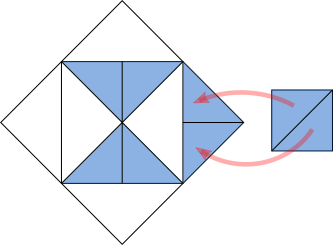#### You may also like### Paving the Way

A man paved a square courtyard and then decided that it was too small. He took up the tiles, bought 100 more and used them to pave another square courtyard. How many tiles did he use altogether?### Square Areas

Can you work out the area of the inner square and give an explanation of how you did it?### Chess

What would be the smallest number of moves needed to move a Knight from a chess set from one corner to the opposite corner of a 99 by 99 square board?

# Square Within a Square Within...

##### Age 11 to 14 Short Challenge Level:

Splitting the diagram up into congruent shapes
Drawing lines through the corners of the smallest square, and then extending these, lots of the triangles are split in half to create lots of triangles the same size. Then extending the sides of the smaller white square, a pattern of squares becomes visible.The diagram is made up of 4 little squares by 4 little squares, so 16 little squares altogether.

Count the number of squares which are half-shaded. There are 12 little shares which are half-shaded, so the same as 6 shaded squares.

So 6 out of 16 squares are shaded, which is $\frac6{16}=\frac38$ of the diagram.

Finding the relationship between the areas of the squares
Consider one square within a square, tilted in this way. By drawing on the diagonals of the shaded square, 4 smaller squares can be formed (each shown in a different colour on the right). Half of each of these squares is shaded, so the shaded square occupies half of the whole square.Now consider the squares below. The first square is the outline of the diagram. The area of the first blue square is half of the area of the diagram. The area of the next white square is half of the area of the first blue square, so $\frac14$ of the area of the diagram. The area of the second blue square is half of the area of the second white square, so $\frac18$ of the area of the diagram.So the shaded area is $\frac12-\frac14+\frac18=\frac48-\frac28+\frac18=\frac38$ of the area of the diagram.So the fraction of the diagram that is shaded is $\frac38$.